# Solution assignment 13 Quadratic equations (factorizing)

### Assignment 13

Try to solve by factorizing: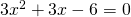### Solution

In this equation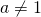, but both sides can be divided by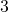, yielding the equation: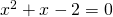Next we have to find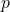and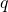such that: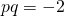and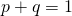The following values will do: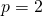and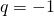Thus the equation can be written as: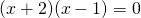and thus the solutions are: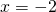or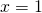0